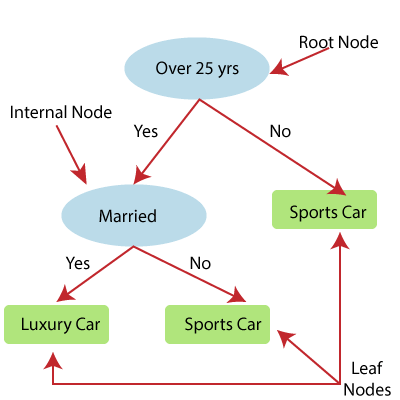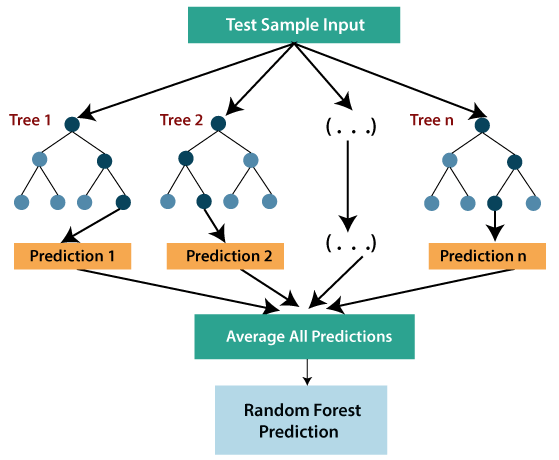# What is Decision Tree Regression?

### Decision Tree Regression:

• Decision Tree is a supervised learning algorithm which can be used for solving both classification and regression problems.
• It can solve problems for both categorical and numerical data
• Decision Tree regression builds a tree-like structure in which each internal node represents the “test” for an attribute, each branch represent the result of the test, and each leaf node represents the final decision or result.
• A decision tree is constructed starting from the root node/parent node (dataset), which splits into left and right child nodes (subsets of dataset). These child nodes are further divided into their children node, and themselves become the parent node of those nodes. Consider the below image:Above image showing the example of Decision Tee regression, here, the model is trying to predict the choice of a person between Sports cars or Luxury car.

• Random forest is one of the most powerful supervised learning algorithms which is capable of performing regression as well as classification tasks.
• The Random Forest regression is an ensemble learning method which combines multiple decision trees and predicts the final output based on the average of each tree output. The combined decision trees are called as base models, and it can be represented more formally as:

g(x)= f0(x)+ f1(x)+ f2(x)+…

• Random forest uses Bagging or Bootstrap Aggregation technique of ensemble learning in which aggregated decision tree runs in parallel and do not interact with each other.
• With the help of Random Forest regression, we can prevent Overfitting in the model by creating random subsets of the dataset.